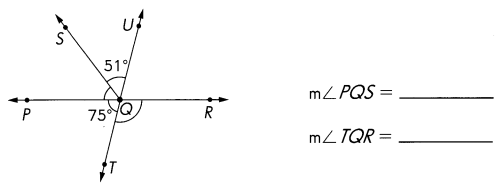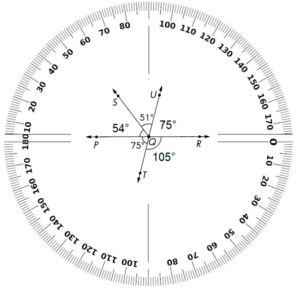# Math in Focus Grade 5 Chapter 12 Practice 2 Answer Key Angles at a Point

This handy Math in Focus Grade 5 Workbook Answer Key Chapter 12 Practice 2 Angles at a Point provides detailed solutions for the textbook questions.

## Math in Focus Grade 5 Chapter 12 Practice 2 Answer Key Angles at a Point

In each figure, the rays meet at a point. Use a protractor to find unknown angle measures.

Question 1.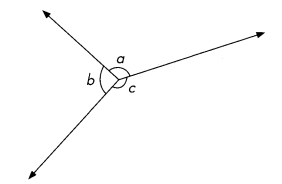m∠a = ___
m∠b = ___
m∠c = ____
m∠a + m∠b + m∠c = ___ + ___ + ___
= ____
m∠a = 120°
m∠b = 90°
m∠c = 150°
m∠a + m∠b + m∠c = 120° + 90° + 150° = 360°
Explanation:
Angle less than 180° is obtuse angle.
m∠a = 120°
m∠c = 150°
Angle 90° is Right angle triangle.
m∠b = 90°
Add all the angles to get a complete angle.
m∠a + m∠b + m∠c = 120° + 90° + 150° = 360°

Question 2.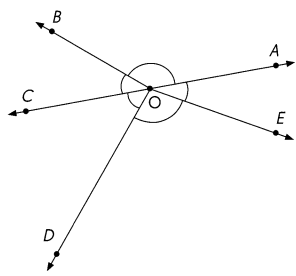m∠AOB = ___
m∠BOC = ___
m∠COD = ____
m∠DOE = _____
m∠AOE = ____
m∠AOB + m∠BOC + m∠COD + m∠DOE + m∠AOE
= ___ + ___ + ___ + ___ + ____
= ____
m∠AOB = 140°
m∠BOC = 40°
m∠COD = 50°
m∠DOE = 100°
m∠AOE = 30°
m∠AOB + m∠BOC + m∠COD + m∠DOE + m∠AOE
= 140°+40°+50°+100°+30°= 360° is called a complete angle.
Explanation: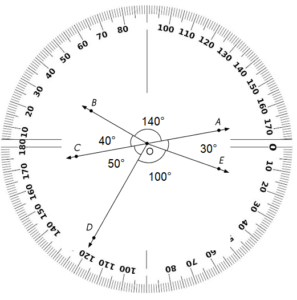m∠AOB = 140°
m∠DOE = 100°
Angle less than 180° is obtuse angle.
m∠BOC = 40°
m∠COD = 50°
m∠AOE = 30°
Angle 90° is Right angle triangle.
Add all the angles to show complete angle.
m∠AOB + m∠BOC + m∠COD + m∠DOE + m∠AOE
= 140°+40°+50°+100°+30°= 360°

Name the angles at a point.

Question 3.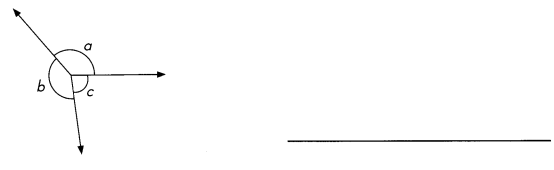∠a = 120°
∠b= 80°
∠c = 160°
∠a + ∠b + ∠c = 120° + 80° + 160° = 360°
Explanation:
∠a = 120° and ∠c = 160° are obtuse angles.
∠b = 80° is acute angle.
∠a + ∠b + ∠c = 120° + 80° + 160° = 360°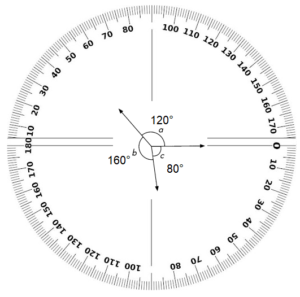Question 4.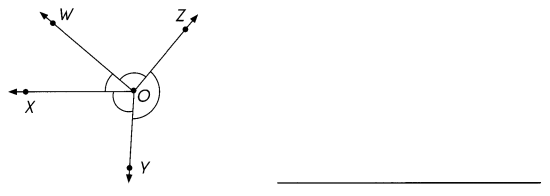m∠XOW = 40°
m∠ZOW = 90°
m∠ZOY = 140°
m∠XOY=90°
m∠XOY + m∠XOW + m∠ZOW + m∠ZOY + m∠XOY
= 40° + 90° + 140° + 90° = 360°
Explanation: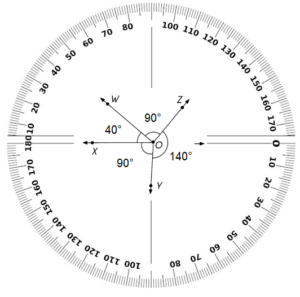In the above picture
m∠ZOW = 90° and m∠XOY=90° are right angles.
m∠XOW = 40° is acute angle as the angle is less than 90°
m∠ZOY = 140° is obtuse angle as the angle is less than 180°
Add all the angles to get complete angle.
m∠XOY + m∠XOW + m∠ZOW + m∠ZOY + m∠XOY
= 40° + 90° + 140° + 90° = 360°

Question 5.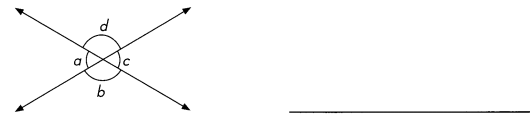∠a = 60°
∠b= 120°
∠c = 60°
∠d= 120°
∠a + ∠b + ∠c +∠d = 60°+ 120° + 60° + 120° = 360°
Explanation: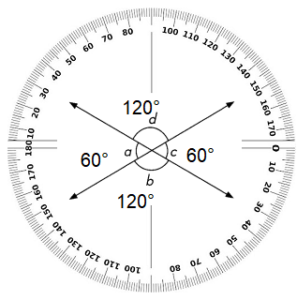As shown in the above picture
∠a + ∠c= 60° are acute angles as the angle is less than 90°
∠b  and ∠d = 120° are obtuse angles as the angle is less than 180°
Add all the angles to get complete angle.
∠a + ∠b + ∠c +∠d = 60°+ 120° + 60° + 120° = 360°

Question 6.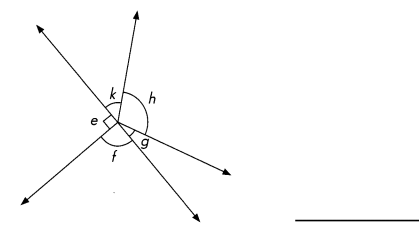∠e = 90°
∠f  = 90°
∠g = 30°
∠h = 100°
∠k = 50°
∠e + ∠f + ∠g +∠h + ∠k =  90° + 90°+ 30° + 100° + 50° = 360°
Explanation: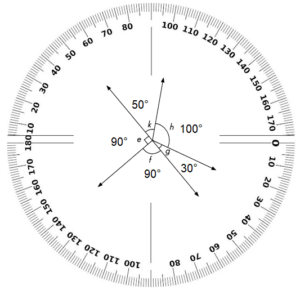As shown in the above picture
∠g = 30° and ∠k= 50° are acute angles as the angle is less than 90°
∠e = 90° and ∠f= 90° are right angles as the angle is 90°
∠h = 100° is obtuse angle as the angle is less than 180°
Add all the angles to get complete angle.
∠e + ∠f + ∠g +∠h + ∠k =  90° + 90°+ 30° + 100° + 50° = 360°

Find the unknown angle measures.

Question 7.
Find the measure of ∠AOB.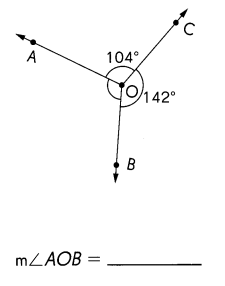Explanation:
complete angle is 360°
∠AOC +∠COB = 104° + 142° = 246°
∠AOB = 360° – 246° = 114°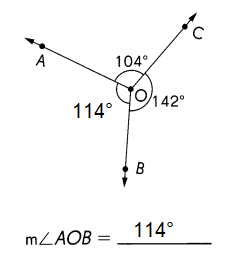Question 8.
Find the measure of ∠a.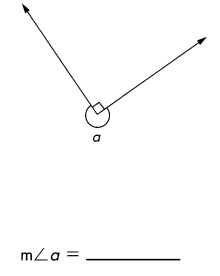Explanation:
complete angle is 360°
360°- 90° = 270°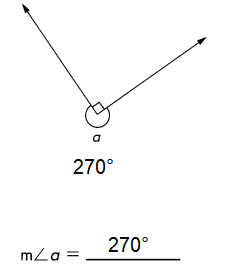Question 9.
Find the measure of ∠b.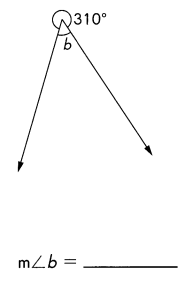Explanation:
complete angle is 360°
360°- 310° = 50°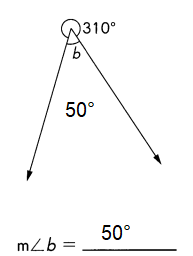Question 10.
Find the measure of ∠c.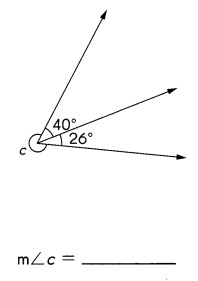Explanation:
complete angle is 360°
given information
40°+ 26° = 66°
360°- 66° = 294°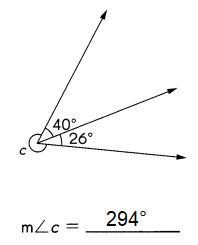Find the unknown angle measures.

Question 11.
Find the measure of ∠q.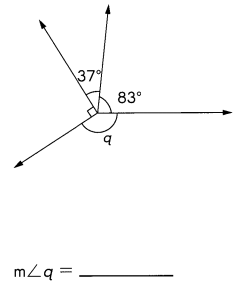Explanation:
complete angle is 360°
given information
90°+37°+ 83° = 210°
360°- 210° = 150°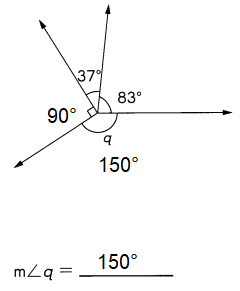Question 12.
Find the measure of ∠r.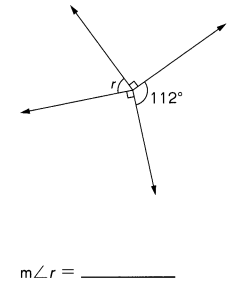Explanation:
complete angle is 360°
given information
90°+90°+ 112° = 292°
360°- 292° = 68°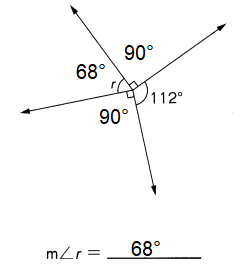Question 13.
Find the measure of ∠a.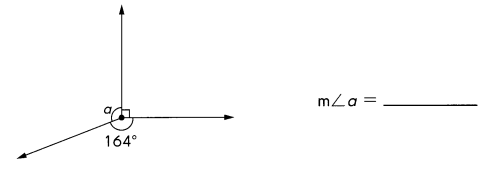Explanation:
complete angle is 360°
given information
90°+164° = 254°
360°- 292° = 106°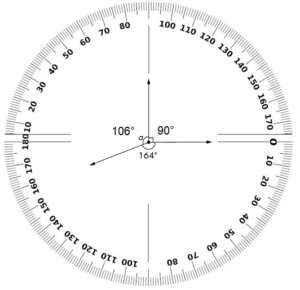Question 14.
$$\overleftrightarrow{P R}$$ and $$\overleftrightarrow{T U}$$ meet at Q. Find the measures of ∠PQSand ∠TQR.